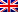The automorphism group of the moduli space of principal bundles on curves

Giovedì 11 Novembre ore 14:00, Roberto Fringuelli (Università degli Studi di Roma "Tor Vergata") terrà il seminario di Geometria dal titolo "The automorphism group of the moduli space of principal bundles on curves".

Abstract: Let G be a complex (connected) almost-simple and simply-connected group and C be a complex smooth projective curve of genus at least three. It is known that the moduli space M_G(C) of semistable G-bundles over C is an irreducible projective variety. The automorphism group of M_G(C) contains the so-called tautological automorphisms: they are induced by the automorphisms of the curve C, outer automorphisms of G and tensorization by Z(G)-torsors, where Z(G) is the center of G. It is a natural question to ask if they generate the entire automorphism group. Kouvidakis and Pantev gave a positive answer when G=SL_n. An alternative proof has been given by Hwang and Ramanan. Later, Biswas, Gomez and Muñoz, after simplifying the proof for G=SL_n, extended the result to the symplectic group Sp(2n). All the proofs rely on the study of the singular fibers of the Hitchin fibration. In this talk, we present a work in progress where, by adapting the Biswas-Gomez-Muñoz strategy, we describe the automorphism group of M_G(C), for any almost-simple and simply-connected group.

Il seminario avrà luogo in presenza presso il Dipartimento di Matematica e Fisica
Lungotevere Dante 376 - Aula M1
This post is also available in: Link identifier #identifier__33948-5Eng